# Excel表格核对的高级技巧并不是用vlookup进行简单的核对

excel表格的核对工作，以前介绍过用vlookup进行简单的核对，今天要分享是一些复杂的核对，这些技巧如果不会，遇到相关问题，花费你的时间可不时一两个小时，所以建议同学们务必要收藏起来这些高级核对技巧。

=IFERROR(LOOKUP(1,0/(B\$1:B1=B2),ROW(\$1:2)),"")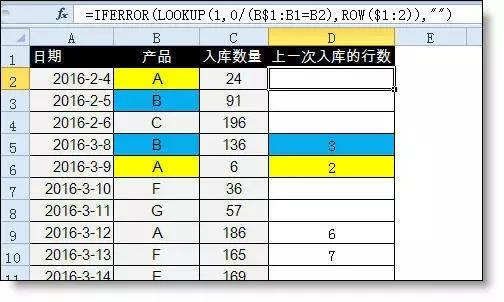=SUMPRODUCT((MONTH(\$A\$3:\$A\$15)=3)*(\$B\$3:\$B\$15=E3)*\$C\$3:\$C\$15)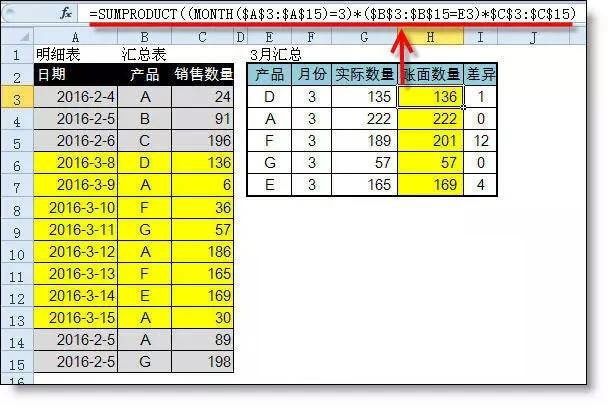【例】如下面的两列数据，需要一对一的金额核对并用颜色标识出来。=COUNTIF(B\$2:B2,B2)&B2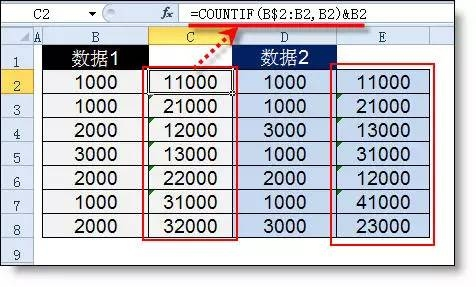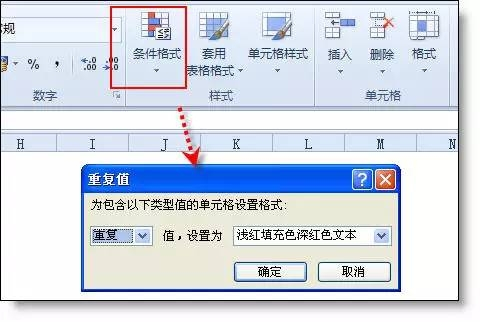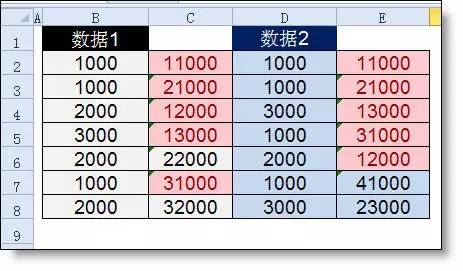C2:

=A2&ABS(B2)&"-"&COUNTIFS(A\$1:A2,A2,\$B\$1:B2,B2)

D2:

=COUNTIF(C:C,C2)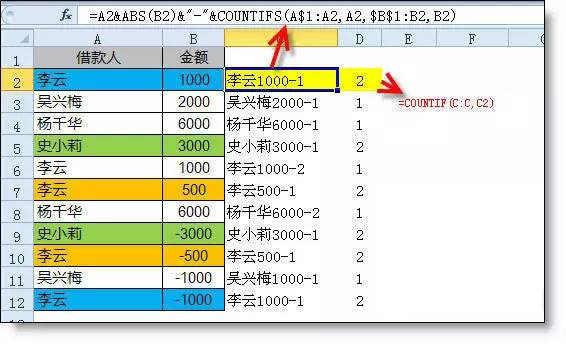tag : 核对   vlookup